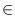Next: Straight Lines in 3D-Space Up: Basic Data Types for Previous: Straight Rays in 3D-Space   Contents   Index

# Segments in 3D-Space ( d3_segment )

Definition

An instance s of the data type d3segment is a directed straight line segment in three-dimensional space, i.e., a straight line segment [p, q] connecting two points p, qR3. p is called the source or start point and q is called the target or end point of s. The length of s is the Euclidean distance between p and q. A segment is called trivial if its source is equal to its target. If s is not trivial, we use line(s) to denote the straight line containing s.

#include < LEDA/geo/d3_segment.h >

Creation

 d3_segment s(const d3_point& p1, const d3_point& p2) introduces a variable s of type d3_segment. s is initialized to the segment from p1 to p2. d3_segment s introduces a variable s of type d3_segment. s is initialized to the segment from (0,0,0) to (1,0,0).

Operations

 bool s.contains(const d3_point& p) decides whether s contains p. d3_point s.source() returns the source point of segment s. d3_point s.target() returns the target point of segment s. double s.xcoord1() returns the x-coordinate of s.source(). double s.xcoord2() returns the x-coordinate of s.target(). double s.ycoord1() returns the y-coordinate of s.source(). double s.ycoord2() returns the y-coordinate of s.target(). double s.zcoord1() returns the z-coordinate of s.source(). double s.zcoord2() returns the z-coordinate of s.target(). double s.dx() returns xcoord2()-xcoord1(). double s.dy() returns ycoord2()-ycoord1(). double s.dz() returns zcoord2()-zcoord1(). segment s.project_xy() returns the projection into the xy plane. segment s.project_xz() returns the projection into the xz plane. segment s.project_yz() returns the projection into the yz plane. d3_segment s.project(const d3_point& p, const d3_point& q, const d3_point& v) returns s projected into the plane through (p,q,v). d3_segment s.reflect(const d3_point& p, const d3_point& q, const d3_point& v) returns s reflected across the plane through (p,q,v). d3_segment s.reflect(const d3_point& p) returns s reflected across point p. d3_segment s.reverse() returns s reversed. vector s.to_vector() returns s.target()-s.source(). bool s.intersection(const d3_segment& t) decides, whether s and t intersect in a single point. bool s.intersection(const d3_segment& t, d3_point& p) decides, whether s and t intersect in a single point. If they intersect in a single point, the point is assigned to p and the result is true, otherwise the result is false bool s.intersection_of_lines(const d3_segment& t, d3_point& p) If line(s) and line(t) intersect in a single point this point is assigned to p and the result is true, otherwise the result is false. bool s.is_trivial() returns true if s is trivial. double s.sqr_length() returns the square of the length of s. double s.length() returns the length of s. d3_segment s.translate(const vector& v) returns s translated by vector v. Precond.: v.dim()=3. d3_segment s.translate(double dx, double dy, double dz) returns s translated by vector (dx,dy,dz). d3_segment s + const vector& v returns s translated by vector v. d3_segment s - const vector& v returns s translated by vector - v.Next: Straight Lines in 3D-Space Up: Basic Data Types for Previous: Straight Rays in 3D-Space   Contents   Index